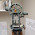## Tuesday, December 16, 2014

### HP Prime and HP 50g: Using the LSQ Function to find the Moore-Penrose Inverse of a Matrix

HP Prime and HP 50g:  Using the LSQ Function to find the Moore-Penrose Inverse of a Matrix

Recall that the Moore-Penrose Inverse of a Matrix A, A^+, with r rows and c columns is:

If r ≤ c and rank(A) = r  (horizontal matrix), then:
A^+ = A^T * (A*A^T)^-1

If r ≥ c and rank(A) = c  (vertical matrix), then:
A^+ = (A^T * A)^-1 * A^T

You can see the blog entry about the Moore-Penrose inverse here:  http://edspi31415.blogspot.com/2014/08/moore-penrose-inverse-of-matrix.html.

On the LSQ (Least Squares Solution Command) function, featured on both the HP Prime and HP 50G, you can find A^+.

Syntax to find A^+ using the LSQ Command

HP Prime:

LSQ(matrix, IDENMAT(r))

Where IDENMAT is the identity matrix command.

Path to IDENMAT:  Toolbox, Math, 7. Matrix, 4. Create, 2. Identity
Path to LSQ:  Toolbox, Math, 7. Matrix, 7. Factorize, 2. LSQ

HP 50g:

2:  identity matrix of dimension r x r  (R IDN)
1:  matrix
LSQ

Build the identity matrix by entering r, then [Left Shift],  (MATRICES), [F1] (CREATE), [F4] (IDN)
Path to LSQ:  [Left Shift],  (MATRICES), [F2] (OPER), [NXT], [F2] (LSQ)

Examples (answers are rounded to five decimal places):

Example 1

M =
[[2, 5],
[3, 3],
[4, 5]]
(3 x 2 matrix, r = 3)

HP Prime:  LSQ(M, IDENMAT(3))
HP 50g:   2:  (3 IDN), 1: M

Result:

M^+ »
[[-0.45026, 0.31579, 0.21579],
[0.35263, -0.15789, -0.05789]]

Example 2

M =
[[4, 0.3, -0.8, 4.8, 2.5],
[-1.8, 3.6, 4.2, 4.4, -7]]
(2 x 5, r = 2)

HP Prime:  LSQ(M, IDENMAT(2))
HP 50g:   2:  (2 IDN), 1: M

Result:

M^+ »
[[0.0853, -0.01272],
[0.01109, 0.03586],
[-0.01224, 0.04039],
[0.11059, 0.04939],
[0.04594, -0.06586]]

This blog is property of Edward Shore. 2014.

#### 1 comment:

1.Thanks Eddie!!!

### Retro Review: Radio Shack EC-2024

Retro Review:  Radio Shack EC-2024 Quick Facts Company:  Radio Shack Years in Production: Around 1994 Original Price: \$24.99 Bat...